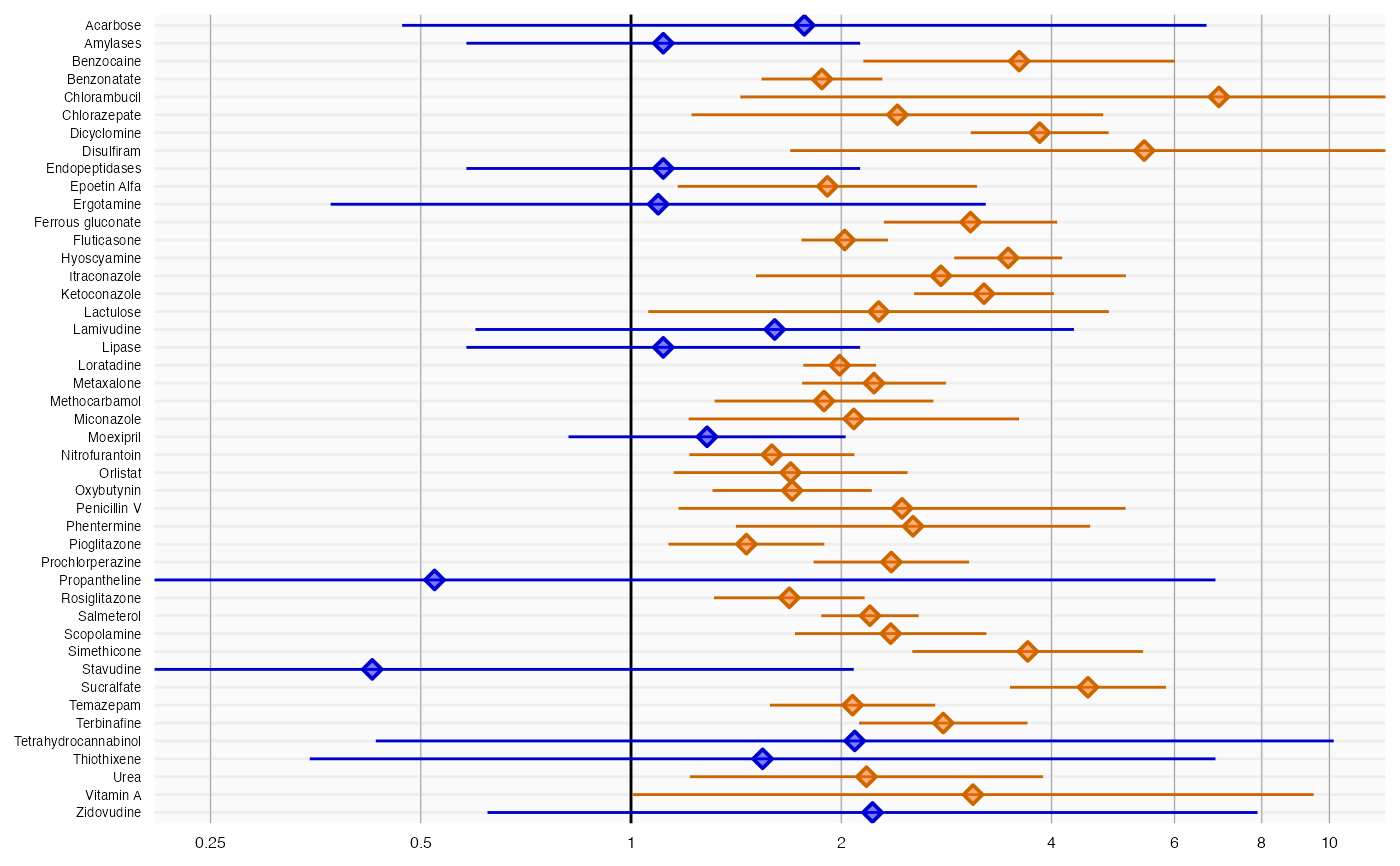plotForest creates a forest plot of effect size estimates.

plotForest(
logRr,
seLogRr,
names,
xLabel = "Relative risk",
title,
fileName = NULL
)

## Arguments

logRr

A numeric vector of effect estimates on the log scale

seLogRr

The standard error of the log of the effect estimates. Hint: often the standard error = (log(<lower bound 95 percent confidence interval>) - log(<effect estimate>))/qnorm(0.025)

names

A vector containing the names of the drugs or outcomes

xLabel

The label on the x-axis: the name of the effect estimate

title

Optional: the main title for the plot

fileName

Name of the file where the plot should be saved, for example 'plot.png'. See the function ggsave in the ggplot2 package for supported file formats.

## Value

A Ggplot object. Use the ggsave function to save to file.

## Details

Creates a forest plot of effect size estimates (ratios). Estimates that are significantly different from 1 (alpha = 0.05) are marked in orange, others are marked in blue.

## Examples

data(sccs)
negatives <- sccs[sccs$groundTruth == 0, ] plotForest(negatives$logRr, negatives$seLogRr, negatives$drugName)